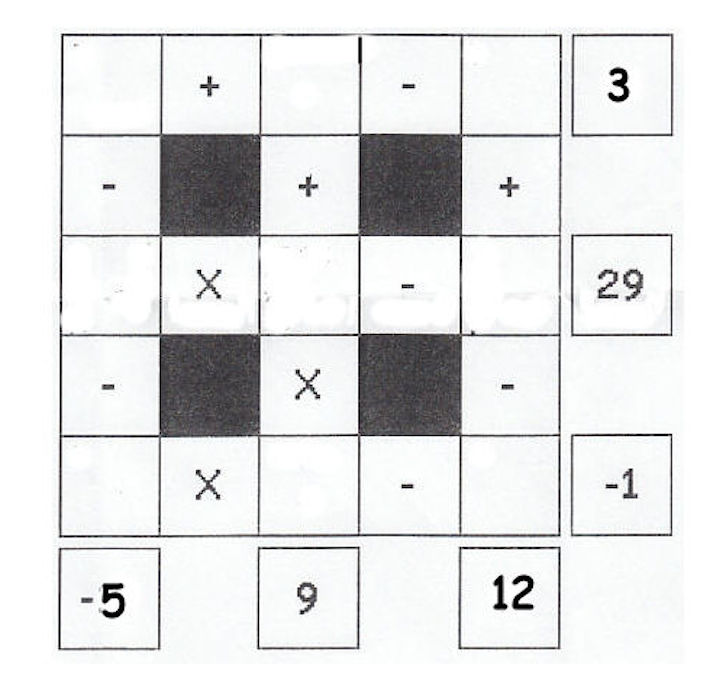# Fill the SquaresFill the empty squares with integers from 1 to 9, using each integer exactly once, to get the given results. Calculations are done from left to right, and from top to bottom.

What is the sum of the sums of numbers on the 2 diagonals?

Note that the center square is added twice.

×